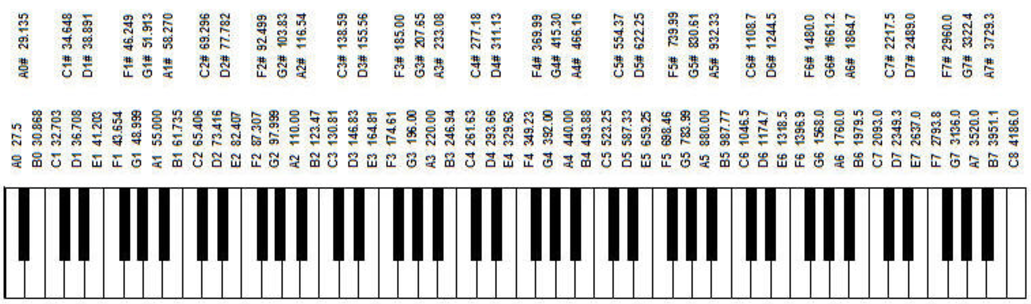# RTTTL Music

## RTTTL¶

While looking into making our Raspberry Pi sing we stumbled across Ring Tone Transfer Language (RTTTL).

RTTTL is a format which specifies each note as a combination of three attributes, the duration, the pitch, and the octave. Here’s an example:

In :
mozart = "Mozart:d=16,o=5,b=125:16d#,c#,c,c#,8e,8p,f#,e,d#,e,8g#,8p,a,g#,g,g#,d#6,c#6,c6,c#6,d#6,c#6,c6,c#6,4e6,8c#6,8e6,32b,32c#6,d#6,8c#6,8b,8c#6,32b,32c#6,d#6,8c#6,8b,8c#6,32b,32c#6,d#6,8c#6,8b,8a#,4g#,d#,32c#,c,c#,8e,8p,f#,e,d#,e,8g#,8p,a,g#,g,g#,d#6,c#6,c6,c#6,d#6,c#6,c6,c#6,4e6,8c#6,8e6,32b,32c#6,d#6,8c#6,8b,8c#6,32b,32c#6,d#6,8c#6,8b,8c#6,32b,32c#6,d#6,8c#6,8b,8a#,4g#"
print(mozart)

Mozart:d=16,o=5,b=125:16d#,c#,c,c#,8e,8p,f#,e,d#,e,8g#,8p,a,g#,g,g#,d#6,c#6,c6,c#6,d#6,c#6,c6,c#6,4e6,8c#6,8e6,32b,32c#6,d#6,8c#6,8b,8c#6,32b,32c#6,d#6,8c#6,8b,8c#6,32b,32c#6,d#6,8c#6,8b,8a#,4g#,d#,32c#,c,c#,8e,8p,f#,e,d#,e,8g#,8p,a,g#,g,g#,d#6,c#6,c6,c#6,d#6,c#6,c6,c#6,4e6,8c#6,8e6,32b,32c#6,d#6,8c#6,8b,8c#6,32b,32c#6,d#6,8c#6,8b,8c#6,32b,32c#6,d#6,8c#6,8b,8a#,4g#


The first three things, “d=8,o=5,b=112” specify the default duration, default octave, and the tempo in beats per minute.

Then come the notes, separated by commas. Notes like “32c#6” have all three attributes: 32 means it’s played for 1/32 times the duration of a whole note, “c#” is the pitch, and 6 means it’s played on the 6th octave (described below).

Notes like “8e” take the default octave, i.e. 5.

Notes like “c#6” take the default duration 8 (1/8th the duration of a whole note).

Notes like “a” take both the defaults.

p”s are pauses.

.”s multiply the duration by 1.5.

Now we can parse a note, given the defaults in a dictionary, as follows:

In :
import re
import numpy as np
from scipy.signal import kaiser
import IPython.display as ipyd

In :
def parse_note(note: str, defaults: dict):
add_duration = False
if "." in note:
add_duration = True
note = note.replace(".", "")
# Matches <a number or nothing><1 or more non-digits><a number or nothing>
# re.I turns on the IGNORECASE flag
match = re.match(r"([0-9]*)(\D+)([0-9]*)", note, re.I)
assert match
duration, key, octave = match.groups()
if not len(duration):
duration = defaults["d"]
else:
duration = int(duration)
if add_duration:
duration += duration/2
if not len(octave):
octave = defaults["o"]
else:
octave = int(octave)
return (duration, key, octave)


## Frequencies¶

As far as we understood from the wiki page, the 4th octave in RTTTL goes from A3 to G#4, 5th octave from A5 to G#6 etc.In :
keys = ["a", "a#", "b", "c", "c#", "d", "d#", "e", "f", "f#", "g", "g#"]
key_dict = dict(zip(keys, range(len(keys))))
def key_to_number(key, octave):
num_per_octave = 12
return key_dict[key] + (num_per_octave * (octave - 1)) + 1


The frequency for each note is $440(2^{(key-49)/12}) Hz$ where key is the number of the key on the piano (starting from 1 to 88). 440Hz is A4.

In :
def key_number_to_frequency(key_number):
return 440 * (2**((key_number-49)/12))


## Sine waves¶

A simple sine waveform (ignoring amplitude) is $y = sin(2\pi x \nu t)$ where $\nu$ is the frequency.

In :
def sine_wave(frequency, duration, rate):
return np.sin(np.arange(duration)/rate * 2 * np.pi * frequency)


We use a kaiser smoothing function to smooth the ends of the waves, otherwise there’s some audible clicks every time the note changes.

In :
class SineSong:
def __init__(self, rtttl_string: str, num_samples=50000, rate=40000):
self.rtttl_string = rtttl_string.strip().replace(' ', '')
rtttl_parts = self.rtttl_string.split(":")
self.name = rtttl_parts
defaults_string = rtttl_parts.split(",")
self.defaults = {p.split("="): int(p.split("=")) for p in defaults_string}
self.notes = rtttl_parts.split(",")
self.num_samples = num_samples
self.rate = rate

def sing(self):
song = []
for note in self.notes:
(duration, key, octave) = parse_note(note, self.defaults)
duration = int(self.num_samples/duration)
if key == "p":
tone = np.zeros(duration)
else:
frequency = key_number_to_frequency(key_to_number(key, octave))
tone = kaiser(duration, beta=7) * sine_wave(frequency, duration, self.rate)
song.append(tone)
song = np.concatenate(song, axis=0)
return song


This page has a nice list of RTTTL songs.

In :
rtttl_songs = {}
with open("data/rtttl-music/songs.txt") as f:
for line in f:
sinesong = SineSong(line)
rtttl_songs[sinesong.name] = sinesong


Here’s the Flintstones:

In :
rtttl_songs["Flintstones"].rtttl_string

Out:
'Flintstones:d=8,o=5,b=200:g#,4c#,p,4c#6,a#,4g#,4c#,p,4g#,f#,f,f,f#,g#,4c#,4d#,2f,2p,4g#,4c#,p,4c#6,a#,4g#,4c#,p,4g#,f#,f,f,f#,g#,4c#,4d#,2c#'
In :
ipyd.Audio(rtttl_songs["Flintstones"].sing(), rate=rtttl_songs["Flintstones"].rate)

Out:

## Raspberry Pi edition¶

Not much extra to get the Pi to play it - we just change the frequency of the piezo buzzer every time the note changes, and sleep during pauses.

In [ ]:
from RPi import GPIO
from time import sleep

class PiSong:
def __init__(self, rtttl_string: str, pin_number, num_samples=50000, rate=40000):
self.rtttl_string = rtttl_string.strip().replace(' ', '')
rtttl_parts = self.rtttl_string.split(":")
self.name = rtttl_parts
defaults_string = rtttl_parts.split(",")
self.defaults = {p.split("="): int(p.split("=")) for p in defaults_string}
self.notes = rtttl_parts.split(",")
self.num_samples = num_samples
self.rate = rate
self.pin_number = pin_number

def sing(self):
GPIO.setmode(GPIO.BOARD)
GPIO.setup(self.pin_number, GPIO.OUT)
pwm = GPIO.PWM(self.pin_number, 1)
pwm.start(1)
for note in self.notes:
(duration, key, octave) = parse_note(note, self.defaults)
duration = int(self.num_samples/duration)
if key == "p":
pwm.stop()
sleep(duration)
else:
frequency = key_number_to_frequency(key_to_number(key, octave))
pwm.start(1)
pwm.ChangeFrequency(frequency)
sleep(duration)

pin_number = 12
pi = PiMusic(rtttl_songs["Flintstones"].rtttl_string, pin_number)
pi.sing()


For comments, click the arrow at the top right corner.